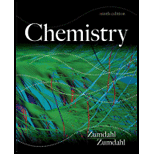# For the reaction 2 H 2 O ( g ) ⇌ 2 H 2 ( g ) + O 2 ( g ) K = 2.4 × 10 −3 at a given temperature. At equilibrium in a 2.0-L container it is found that [H 2 O ( g )] = 1.1 × 10 − 1 M and [H 2 ( g )] = 1.9 × 10 − 2 M. Calculate the moles of O 2 ( g ) present under these conditions.### Chemistry

9th Edition
Steven S. Zumdahl
Publisher: Cengage Learning
ISBN: 9781133611097### Chemistry

9th Edition
Steven S. Zumdahl
Publisher: Cengage Learning
ISBN: 9781133611097

#### Solutions

Chapter
Section
Chapter 13, Problem 43E
Textbook Problem

## Expert Solution

### Want to see the full answer?

Check out a sample textbook solution.See solution

### Want to see this answer and more?

Experts are waiting 24/7 to provide step-by-step solutions in as fast as 30 minutes!*

See Solution

*Response times vary by subject and question complexity. Median response time is 34 minutes and may be longer for new subjects.